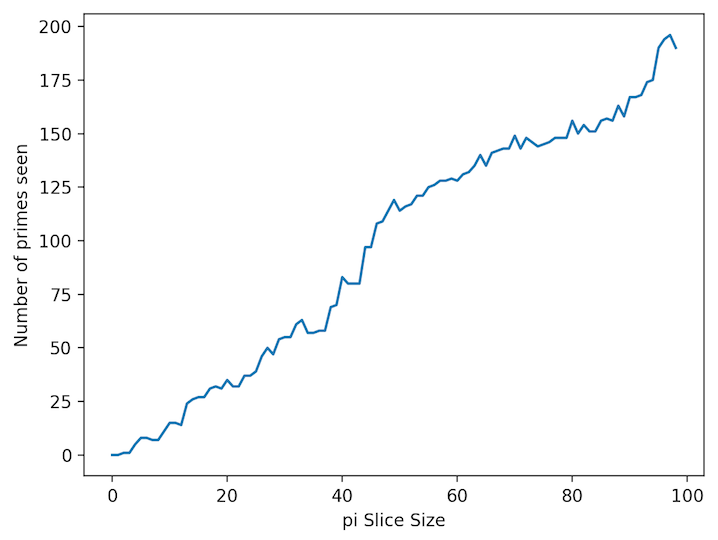# How Many Primes Are In A Slice Of Pi?

Questions:

• What is the number of primes per n-slice of the decimal part of pi?
• What does the graph look like?
• Are there many overlapping primes (i.e. start at the same position in the slice)?

The number of primes increases for each decimal-slice of pi.  For a slice of size one, that is the digit "1" (the first digit of the decimal "1415926536..."), there are no primes.  Easy enough.  For 2-slice the digits are "14" and again there are no primes.  For the slice of 3 digits we get "142" (that's the decimal rounded at the 3rd place!), and there is one prime found - the "2."  And so on... At 10 digits there are 11 primes. At 50 digits there are 119 primes.  A graph will illustrate better. :-)As for prime overlaps, if we look at 500 digits, there are 3 primes that begin at the first position - 14159, 141592653589 and a number with 281 digits - whew!  There are 5 at the second position, 2 at the third, etc.  Again, a graph will help show the results:This shows a decreasing number of overlaps as the position increases, because a smaller and smaller sized slice is used as we reach the end.

The code for this can be found here: pi-slice.py